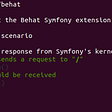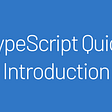# The reason for writing a parser

`selector: bodyproperties:  title: title  articles:    selector: article {0,}    properties:      body: .body::property(innerHTML)      summary: .body p {0,}      imageUrl: img::attribute(src)      title: .title::text()::test(/foo/)::format(upperCase)`

# Picking the right tool for the jobWho needs all of these other parsers when there is He-Man.

# Setup

`\$ npm install nearley`
`\$ ls -1 ./node_modules/.binnearley-railroadnearley-testnearley-unparsenearleyc`
• `nearley-railroad` is used to generate railroad diagrams.
• `nearley-test` is used to test an arbitrary input against the compiled grammar.
• `nearley-unparse` is used to generate random strings that satisfy the grammar.
• `nearleyc` is used to compile Nearley grammar to JavaScript script.

# Parsing “1+2+3”

`expression -> "1+2+3"`

# Testing grammar

`\$ cat <<'EOF' > ./grammar.neexpression -> "1+2+3"EOF\$ nearleyc ./grammar.ne --out ./grammar.js`
`nearley-test ./grammar.js --input '1+2+3'Table length: 6Number of parses: 1Parse ChartsChart: 00: {expression →  ● expression\$string\$1}, from: 01: {expression\$string\$1 →  ● "1" "+" "2" "+" "3"}, from: 0Chart: 10: {expression\$string\$1 → "1" ● "+" "2" "+" "3"}, from: 0Chart: 20: {expression\$string\$1 → "1" "+" ● "2" "+" "3"}, from: 0Chart: 30: {expression\$string\$1 → "1" "+" "2" ● "+" "3"}, from: 0Chart: 40: {expression\$string\$1 → "1" "+" "2" "+" ● "3"}, from: 0Chart: 50: {expression\$string\$1 → "1" "+" "2" "+" "3" ● }, from: 01: {expression → expression\$string\$1 ● }, from: 0Parse results:[ [ '1+2+3' ] ]`

# Table of partial parsings

`Chart: 00: {expression →  ● expression\$string\$1}, from: 01: {expression\$string\$1 →  ● "1" "+" "2" "+" "3"}, from: 0`
`Chart: 10: {expression\$string\$1 → "1" ● "+" "2" "+" "3"}, from: 0Chart: 20: {expression\$string\$1 → "1" "+" ● "2" "+" "3"}, from: 0Chart: 30: {expression\$string\$1 → "1" "+" "2" ● "+" "3"}, from: 0Chart: 40: {expression\$string\$1 → "1" "+" "2" "+" ● "3"}, from: 0Chart: 50: {expression\$string\$1 → "1" "+" "2" "+" "3" ● }, from: 0`
`1: {expression → expression\$string\$1 ● }, from: 0Parse results:[ [ '1+2+3' ] ]`
`expression ->    "1+2+0"  | "1+2+3"`

# Postprocessors

`[  [    '1+2+3'  ]]`
`expression ->  "1" "+" "2" "+" "3"`
`[  [    '1',    '+',    '2',    '+',    '3'  ]]`

# Seriously, whats a postprocessor?

`expression ->  "1" "+" "2" "+" "3" {% d => d.join('') %}EOF`
`[  '1+2+3']`

# Using a nonterminal inside a nonterminal

`expression ->  N MS N MS N  {% d => d.join('') %}MS ->    "+" {% d => d %}  | "-" {% d => d %}N ->    "1" {% d => d %}  | "2" {% d => d %}  | "3" {% d => d %}  | "4" {% d => d %}  | "5" {% d => d %}  | "6" {% d => d %}  | "7" {% d => d %}  | "8" {% d => d %}  | "9" {% d => d %}  | "0" {% d => d %}`

# Recursive patterns

## Once or more

`N ->    "0" {% d => d %}  | "1" {% d => d %}  | "2" {% d => d %}  | "3" {% d => d %}  | "4" {% d => d %}  | "5" {% d => d %}  | "6" {% d => d %}  | "7" {% d => d %}  | "8" {% d => d %}  | "9" {% d => d %}  | "0" N {% d => d + d %}  | "1" N {% d => d + d %}  | "2" N {% d => d + d %}  | "3" N {% d => d + d %}  | "4" N {% d => d + d %}  | "5" N {% d => d + d %}  | "6" N {% d => d + d %}  | "7" N {% d => d + d %}  | "8" N {% d => d + d %}  | "9" N {% d => d + d %}`

## Zero or more

`N ->    null  | "0" N {% d => d + d %}  | "1" N {% d => d + d %}  | "2" N {% d => d + d %}  | "3" N {% d => d + d %}  | "4" N {% d => d + d %}  | "5" N {% d => d + d %}  | "6" N {% d => d + d %}  | "7" N {% d => d + d %}  | "8" N {% d => d + d %}  | "9" N {% d => d + d %}`

# Character sets and quantifiers

`N ->  [0-9]:+ {% d => d.join('') %}`
`N ->  [0-9]:* {% d => d.join('') %}`

# Recap

• what is a nonterminal
• what is a terminal
• what is a production rule
• how to debug grammar
• what is a `nearley` postprocessor
• what are character sets
• what are quantifiers
• what is a BNF recursive repeat construct

# Parsing (and evaluating) mathematical expressions

`main -> AS {% d => d %}# ParenthesesP -> "(" AS ")" {% d => d %}    | N {% d => d %}# Multiplication and divisionMD -> MD "*" P {% d => d*d %}    | MD "/" P {% d => d/d %}    | P {% d => d %}# Addition and subtractionAS ->    AS "+" MD {% d => d+d %}  | AS "-" MD {% d => d-d %}  | MD {% d => d %}N ->    [0-9]:+ {% d => parseInt(d.join(''), 10) %}`

# Ambiguity

`[  [    '1+2+3'  ]]`
`[  '1+2+3']`
`attributeName ->  [_a-zA-Z]:* [_a-zA-Z0-9-]:* {% d => d.join('') + d.join('') %}`
`[  'foobar',  'foobar']`
`attributeName ->  [_a-zA-Z] [_a-zA-Z0-9-]:* {% d => d + d.join('') %}`

# Rejecting a match

• The first parameter is a list of the matched tokens.
• The second is the index at which the rule was found.
• The third is a sentinel value used to reject the match.
`selectorBody ->  typeSelector:?  idSelector:?  classSelector:*  attributeValueSelector:*  attributePresenceSelector:*  pseudoClassSelector:* {%d flatten %d}`
• a type selector (optional)
• an id selector (optional)
• multiple class selectors (optional)
• multiple attribute value selectors (optional)
• multiple presence selectors and (optional)
• multiple pseudo class electors (optional)
`selectorBody ->    TS   IS:? CS:* AVS:* APS:* PCS:* {% flatten %}  | TS:? IS   CS:* AVS:* APS:* PCS:* {% flatten %}  | TS:? IS:? CS   AVS:* APS:* PCS:* {% flatten %}  | TS:? IS:? CS:* AVS   APS:* PCS:* {% flatten %}  | TS:? IS:? CS:* AVS:* APS   PCS:* {% flatten %}  | TS:? IS:? CS:* AVS:* APS:* PCS   {% flatten %}`
`selectorBody ->    TS:? IS:? CS:* AVS:* APS:* PCS:* {% (d, i, reject) => { const tokens = d.filter((token) => { return token !== null; }); if (!tokens.length) return reject; return flatten(tokens); } %}`

# Helper functions

`@{%  const flatten = d => {    return d.reduce(      (a, b) => {        return a.concat(b);      },      []    );  };const filter = d => {    return d.filter((token) => {      return token !== null;    });  };const selectorBody = (d, i, reject) => {    const tokens = filter(d);if (!tokens.length) {      return reject;    }return flatten(tokens);  }%}selectorBody ->    TS:? IS:? CS:* AVS:* APS:* PCS:* {% selectorBody %}`

# Parsing and tokenising

`N ->    [0-9]:+ {% d => {type: 'NUMBER', value: parseInt(d.join(''), 10)} %}`

# Bonus: IDE grammar

• Atom users can write nearley grammars with this plugin by Bojidar Marinov.
• Sublime Text users can write nearley grammars with this syntax by liam4.
• Vim users can use this plugin by Andrés Arana.

# The CSS selector parser

--

--

--

## More from Gajus Kuizinas

Tech / Product Founder — building https://contra.com/

Love podcasts or audiobooks? Learn on the go with our new app.

## Setup Behat with Symfony 5 Rest API## 10 Random topics from React.js## PHP VS NODE.JS — Which One is Better for You?## How JavaScript Engines Work## Let’s build a React app in TDD## Organize NodeJS code using custom modules## Gajus Kuizinas

Tech / Product Founder — building https://contra.com/

## LeetCode’s Combinations Problem- Recursive Backtracking in JavaScript## Typescript Quick Introduction## HackerRank-Easy-Staircase(Typescript)## .map(), .filter() and .reduce() in JavaScript